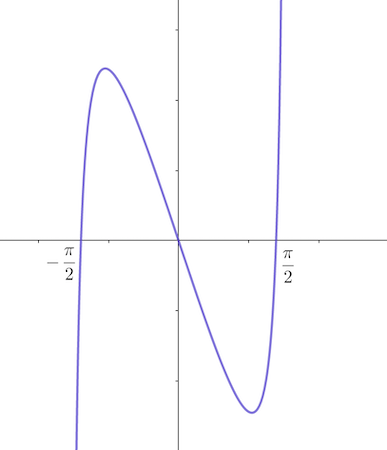# For the function f (x) = tan(x) - 4x on the interval (-\pi/2, \pi/2), determine the critical...

## Question:

For the function {eq}f (x) = tan(x) - 4x {/eq} on the interval ({eq}-\pi/2, \pi/2 {/eq}), determine the critical points, on which intervals {eq}f(x) {/eq} is increasing, on which intervals {eq}f(x) {/eq} is decreasing, on which intervals {eq}f(x) {/eq} is concave up, and on which intervals {eq}f(x) {/eq} is concave down. Find the local extrema using the second derivative test. Use this information to sketch the graph.

## Sketching Graphs

To see where a function {eq}\displaystyle y=f(x) {/eq} is increasing or decreasing, we will look at the sign of its first derivative: {eq}\displaystyle \text{ if } f'(x)>0 \text{ then the function is increasing} \displaystyle \text{ and if } f'(x)<0 \text{ the function is decreasing}. {/eq}

The concavity of a function, when the graph is not given, is determined by the second derivative test.

{eq}\displaystyle \text{ if } f''(x)>0 \implies f(x) \text{ is concave up}\\ \displaystyle \text{ if } f''(x)<0 \implies f(x) \text{ is concave down}. {/eq}

We can determine the local extreme points by the analyzing how the sign of the first derivative changes, or the sign of the second derivative.

If the first derivative changes from positive to negative or the second derivative is negative, then the point is a local maximum.

To determine the interval where the function {eq}\displaystyle f(x)= \tan x-4x, \ \left(-\frac{\pi}{2}, \frac{\pi}{2}\right) {/eq} is increasing/decreasing, concave upward / downward,

we will first obtain the first and second derivatives.

{eq}\displaystyle \begin{align} f'(x)&= \frac{d}{dx}\left( \tan x-4x\right)\\ &=\sec^2 x -4, &\left[\text{using } \frac{d}{dx}(\tan x)=\sec^2 x \right]\\ \implies &\text{the first derivative zeros are obtained from } \sec x=\pm 2\implies x=\pm \frac{\pi}{3} \text{ in } \left(-\frac{\pi}{2}, \frac{\pi}{2}\right)\\ \implies &\text{ because } f'(0)=-4<0\implies \boxed{\text{ the function is increasing on } \left(-\frac{\pi}{2}, -\frac{\pi}{3}\right) \cup \left(\frac{\pi}{3}, \frac{\pi}{2}\right)},\\ &\text{ and the function is decreasing on }\left(-\frac{\pi}{3}, \frac{\pi}{3}\right) .\\ &\text{when the derivative changes sings from negative to positive, the point is a local minimum }\\ \implies &\boxed{\text{ the point } -\frac{\pi}{3} \text{ is a local maximum, and the point } \frac{\pi}{3} \text{ is a local minimum }}. \end{align} {/eq}

The second derivative,

{eq}\displaystyle \begin{align} f''(x)&=\frac{d}{dx}\left( \frac{df}{dx}\right)\\ &=\frac{d}{dx}\left(\sec^2 x -4\right)\\ &=2 \sec^2 x \tan x, &\left[\text{using Chain rule and } \frac{d}{dx}(\sec x)=\sec x\tan x \right]\\ &\text{ the second derivative is positive when } \tan x< 0, & \text{ because the other factor, } \sec^2 x \text{ is positive everywhere }\\ \iff &\boxed{\text{ the function is concave downward on } \left(-\frac{\pi}{2}, 0\right) \text{ and is concave upward on } \left(0, \frac{\pi}{2}\right)}. \end{align} {/eq}

Below, we plotted the function with solid blue line,